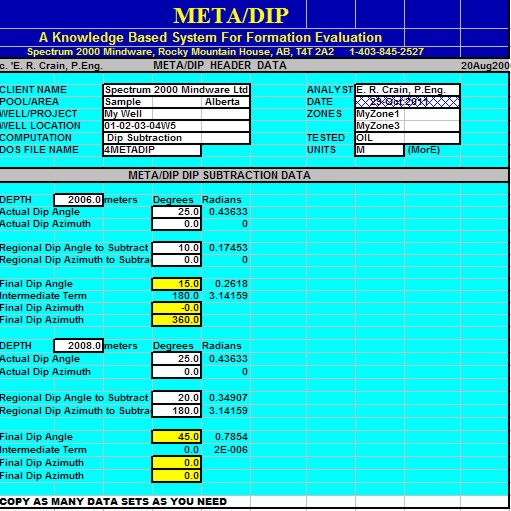Dip Subtraction and Rotation
Dip subtraction, also called dip rotation, is used to translate actual dip to dip with regional dip removed. The result is used to assess the actual angles of crossbedding or fault planes relative to horizontal strata. If you do not have a dip removed arrow plot, you may have to perform this calculation on a few dips to find depositional dip patterns. The equations are:
1: NEWDIP = Arccos(Cos SD * Cos DIP + Sin SD * Sin DIP * Cos(AZM - SDAZ))
2: ANGLS = Arccos((Cos DIP - Cos SD * Cos NEWDIP) / (Sin SD * Sin NEWDIP))
3: IF Sin (AZM - SDAZ) >= 0
4: THEN NEWAZM = SDAZ + 180 - ANGLS
5: Otherwise NEWAZM = SDAZ - 180 + ANGLS
6: NEWAZM = 360 * Frac((NEWAZM + 360) / 360)

In a July 2017 email, Charles Berg at ResDip pointed out that NEWDIP could exceed 90 degrees and that we usually do not report dips this way. An additional step provided by Charles corrects this problem:
7: IF NEWDIP > 90
8: THEN NEWDIP = 180 - NEWDIP
9: AND NEWAZM = 180 + NEWAZM

Where:
ANGLS = intermediate term
AZM = true dip azimuth before structural dip removal
DIP = true dip angle before structural dip removal
NEWDIP = dip after structural dip removal
NEWAZM = azimuth after structural dip removal
SD = structural (regional) dip to remove
SDAZ = azimuth of structural dip

It is sometimes necessary or desirable to project the actual dip onto a new azimuth. This "apparent dip" calculation is used to prepare dips for presentation on a stick diagram at arbitrary cross section orientations, such as the line of section between two wells, or along the section of a seismic line. The equation is:
10: PROJDIP = Arctan (Tan DIP * Cos (PROJAZM - AZM))

Where:
AZM = true dip azimuth before rotation
DIP = true dip angle before rotation
PROJDIP = projected dip
PROJAZM = projected azimuthMETALOG "/DIP" SPREADSHEET--Spreadsheet for Dip Subtraction
This spreadsheet provides a tool for Dip Subtraction (Regional Dip Removal) and Dip (Apparent Dip in any arbitrary azimuth). Dip azimuth is trimmed to within a 360 degree compass.

SPR-25 META/LOG DIP SUBTRACTION CALCULATOR
Regional dip removal -- dip subtraction.Example from META/LOG "DIP" spreadsheet for dip subtraction or rotation.

Page Views ---- Since 01 Jan 2015
Copyright 2023 by Accessible Petrophysics Ltd.
CPH Logo, "CPH", "CPH Gold Member", "CPH Platinum Member", "Crain's Rules", "Meta/Log", "Computer-Ready-Math", "Petro/Fusion Scripts" are Trademarks of the Author